# Resources tagged with: Constructions

Filter by: Content type:
Age range:
Challenge level:

### There are 22 results

Broad Topics > Transformations and constructions > Constructions### Close to Triangular

##### Age 14 to 16 Challenge Level:

Drawing a triangle is not always as easy as you might think!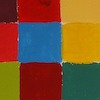### The Square Under the Hypotenuse

##### Age 14 to 16 Challenge Level:

Can you work out the side length of a square that just touches the hypotenuse of a right angled triangle?### Squirty

##### Age 14 to 16 Challenge Level:

Using a ruler, pencil and compasses only, it is possible to construct a square inside any triangle so that all four vertices touch the sides of the triangle.### Three Tears

##### Age 14 to 16 Challenge Level:

Construct this design using only compasses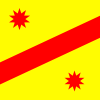### Two Points Plus One Line

##### Age 14 to 16 Challenge Level:

Draw a line (considered endless in both directions), put a point somewhere on each side of the line. Label these points A and B. Use a geometric construction to locate a point, P, on the line,. . . .### Moving Squares

##### Age 14 to 16 Challenge Level:

How can you represent the curvature of a cylinder on a flat piece of paper?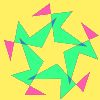### Attractive Rotations

##### Age 11 to 14 Challenge Level:

Here is a chance to create some attractive images by rotating shapes through multiples of 90 degrees, or 30 degrees, or 72 degrees or...### The Farmers' Field Boundary

##### Age 11 to 14 Challenge Level:

The farmers want to redraw their field boundary but keep the area the same. Can you advise them?### LOGO Challenge 8 - Rhombi

##### Age 7 to 16 Challenge Level:

Explore patterns based on a rhombus. How can you enlarge the pattern - or explode it?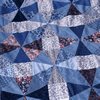### Mathematical Patchwork

##### Age 7 to 14

Jenny Murray describes the mathematical processes behind making patchwork in this article for students.### Circle Scaling

##### Age 14 to 16 Challenge Level:

Describe how to construct three circles which have areas in the ratio 1:2:3.### Triangle Midpoints

##### Age 14 to 16 Challenge Level:

You are only given the three midpoints of the sides of a triangle. How can you construct the original triangle?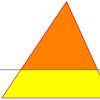### Half a Triangle

##### Age 14 to 16 Challenge Level:

Construct a line parallel to one side of a triangle so that the triangle is divided into two equal areas.### Folding Squares

##### Age 14 to 16 Challenge Level:

The diagonal of a square intersects the line joining one of the unused corners to the midpoint of the opposite side. What do you notice about the line segments produced?### Folding Fractions

##### Age 14 to 16 Challenge Level:

What fractions can you divide the diagonal of a square into by simple folding?### The Medieval Octagon

##### Age 14 to 16 Challenge Level:

Medieval stonemasons used a method to construct octagons using ruler and compasses... Is the octagon regular? Proof please.### Pareq Exists

##### Age 14 to 16 Challenge Level:

Prove that, given any three parallel lines, an equilateral triangle always exists with one vertex on each of the three lines.### Constructing Triangles

##### Age 11 to 14 Challenge Level:

Generate three random numbers to determine the side lengths of a triangle. What triangles can you draw?### LOGO Challenge 2 - Diamonds Are Forever

##### Age 7 to 16 Challenge Level:

The challenge is to produce elegant solutions. Elegance here implies simplicity. The focus is on rhombi, in particular those formed by jointing two equilateral triangles along an edge.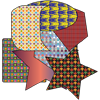##### Age 7 to 14 Challenge Level:

What shape and size of drinks mat is best for flipping and catching?### Curvy Areas

##### Age 14 to 16 Challenge Level:

Have a go at creating these images based on circles. What do you notice about the areas of the different sections?### Cool as Ice

##### Age 11 to 16 Challenge Level:

Design and construct a prototype intercooler which will satisfy agreed quality control constraints.